# SAR成像学习（一）信号到原始数据&原始数据到图像

## 1 信号到复数数据-原始数据是怎么来的

SAR原始数据、SAR信号数据或SAR相位历程是SAR系统通常记录的下传的信号。由于方位向信号常有一个非零的多普勒中心频率，该信号只在距离向上基带信号。

### 1.1 粗略的过程

μ(t)=e j2π(ft+0.5at 2 )

μ(t)=cos[2π(ft+0.5at 2 )]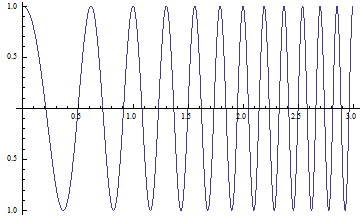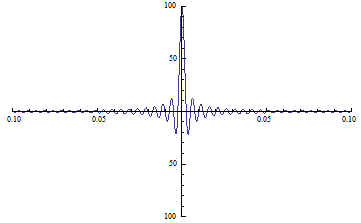2cosαcosβ=cos(αβ)+cos(α+β)

2sinαcosβ=sin(αβ)+sin(α+β)

2sinαsinβ=cos(αβ)cos(α+β)

x(τ)=cos(2πf 0 τ+ϕ(τ))

x c1 (τ)=12 cos(ϕ(τ))+12 cos(4πf 0 τ+ϕ(τ))

x c2 (τ)=12 cos(ϕ(τ))

x s1 (τ)=12 sin(ϕ(τ))+12 sin(4πf 0 τ+ϕ(τ))

x s2 (τ)=12 sin(ϕ(τ))

x 3 (τ)=x c2 (τ)+jx s2 (τ)

### 1.2 结合实际回波信号考虑

ϕ(τ)=4πf 0 R(η)c +πK r [τ2R(η)c ] 2 +ψ

S r (τ,η)=A 0 ω r (τ2R(η)/c)ω a (ηη c )cos(2πf 0 τ+ϕ(τ))

S r (τ,η)=A 0 ω r (τ2R(η)/c)ω a (ηη c )x(τ))

• A 0  $A_0$ 表示后向散射系数的幅度；

• ω r (τ2R(η)/c) $\omega_r (\tau - 2R(\eta) /c)$表示脉冲包络，近似为：
ω r (τ)=rect(τT r  )
其中T r  $T_r$表示脉冲持续时间。
• ω a (ηη c ) $\omega_a (\eta - \eta_{c})$表示随着平台的移动，即η $\eta$的变化，接收到的信号的强度，其中η c  $\eta_{c}$为零多普勒时间,对应多普勒中心频率。

S 0 (τ,η)=A 0 ω r (τ2R(η)/c)ω a (ηη c )exp(ϕ(τ))

S 0 (τ,η)=A 0 ω r (τ2R(η)/c)ω a (ηη c )exp(j4πf 0 R(η)c )exp(jπK r [τ2R(η)c ] 2 )

A 0 =A    0 exp(jψ)

## 2 复数数据聚焦成高分辨率影像-一个示例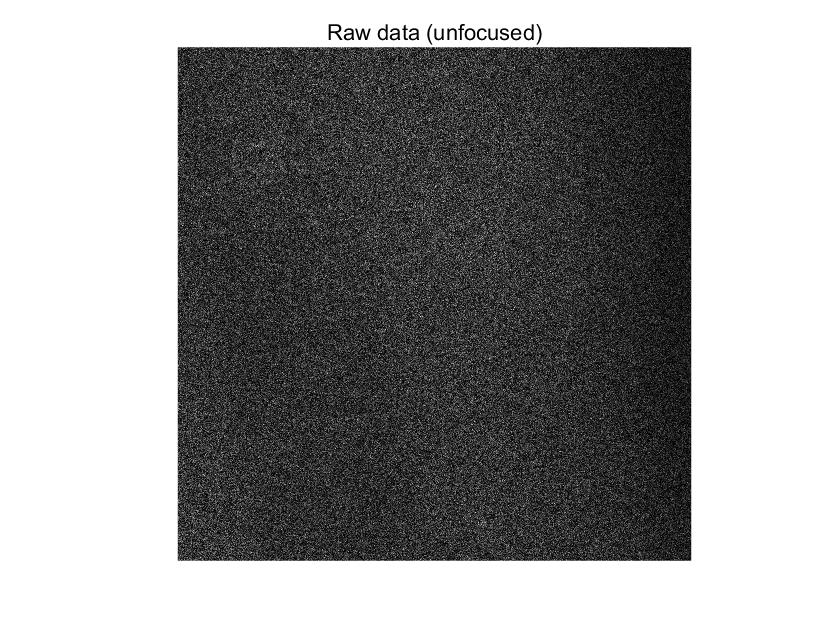unfocused data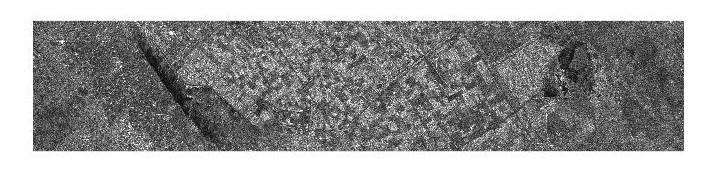focused data

* 计算距离向参考信号，并fft，并共轭；
* 计算方位向参考信号，并fft，并共轭；
* Range compression
* Azimuth compression
* Spatial Multilooking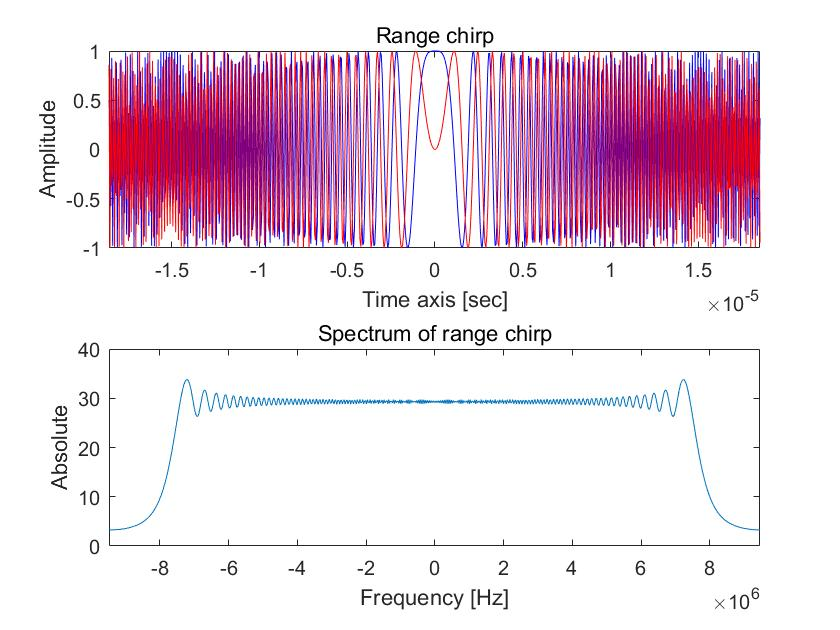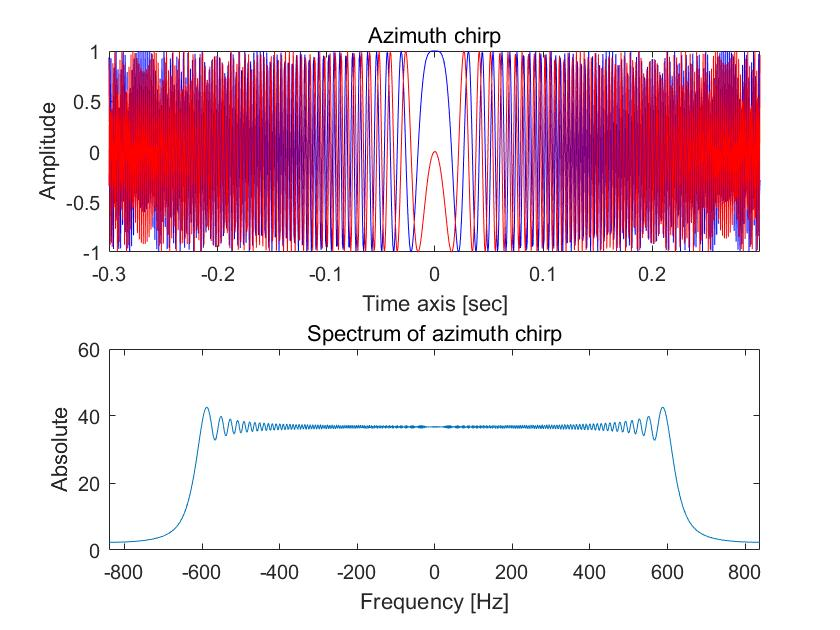## 3 主要参考和感谢

Digital Processing of Synthetic Aperture Radar Data: Algorithms and Implementation

https://saredu.dlr.de